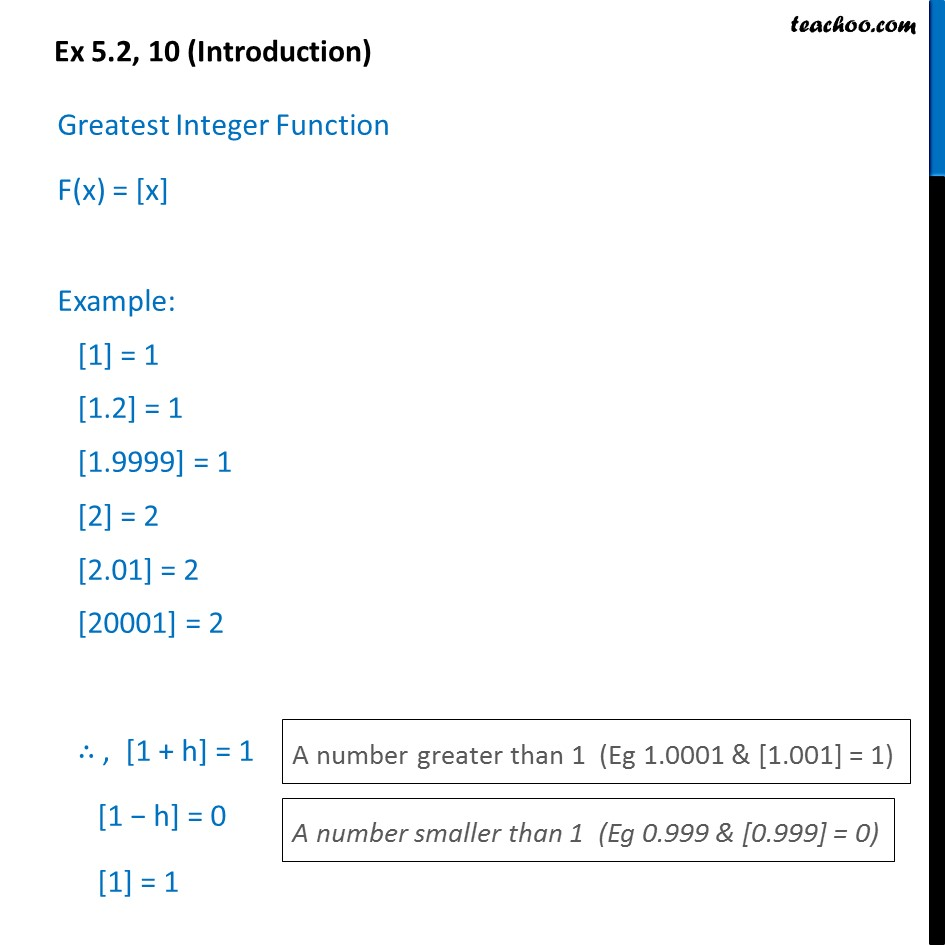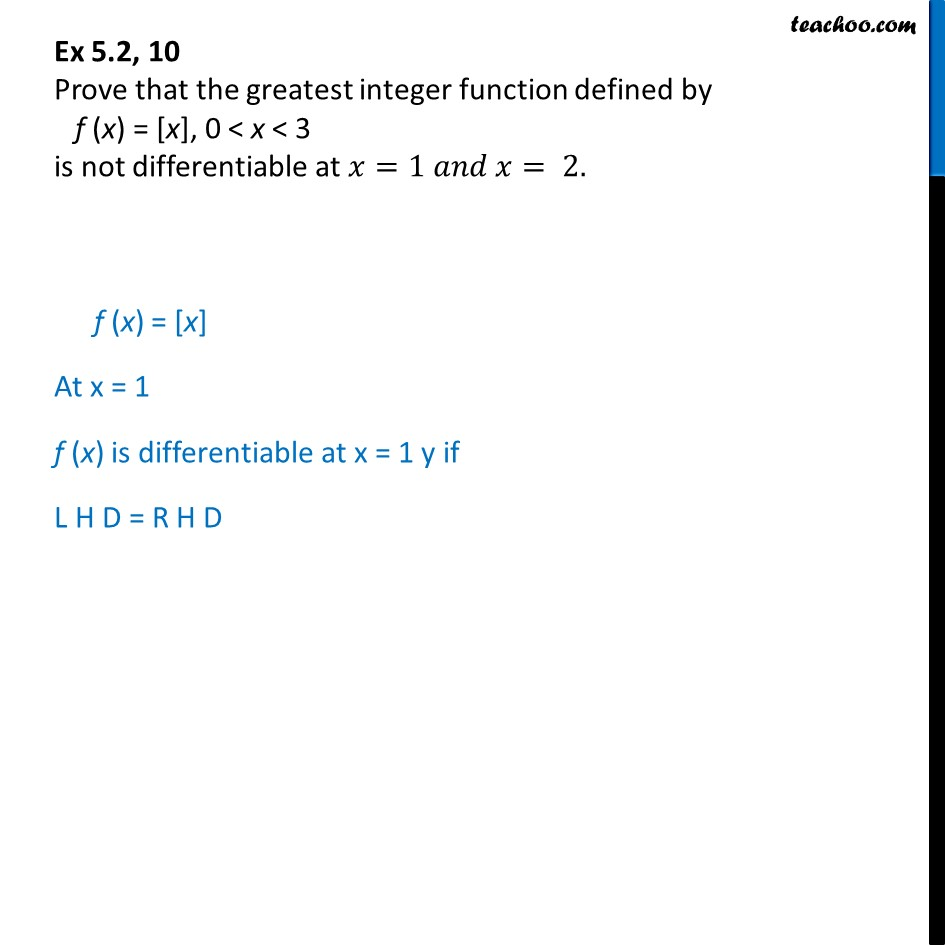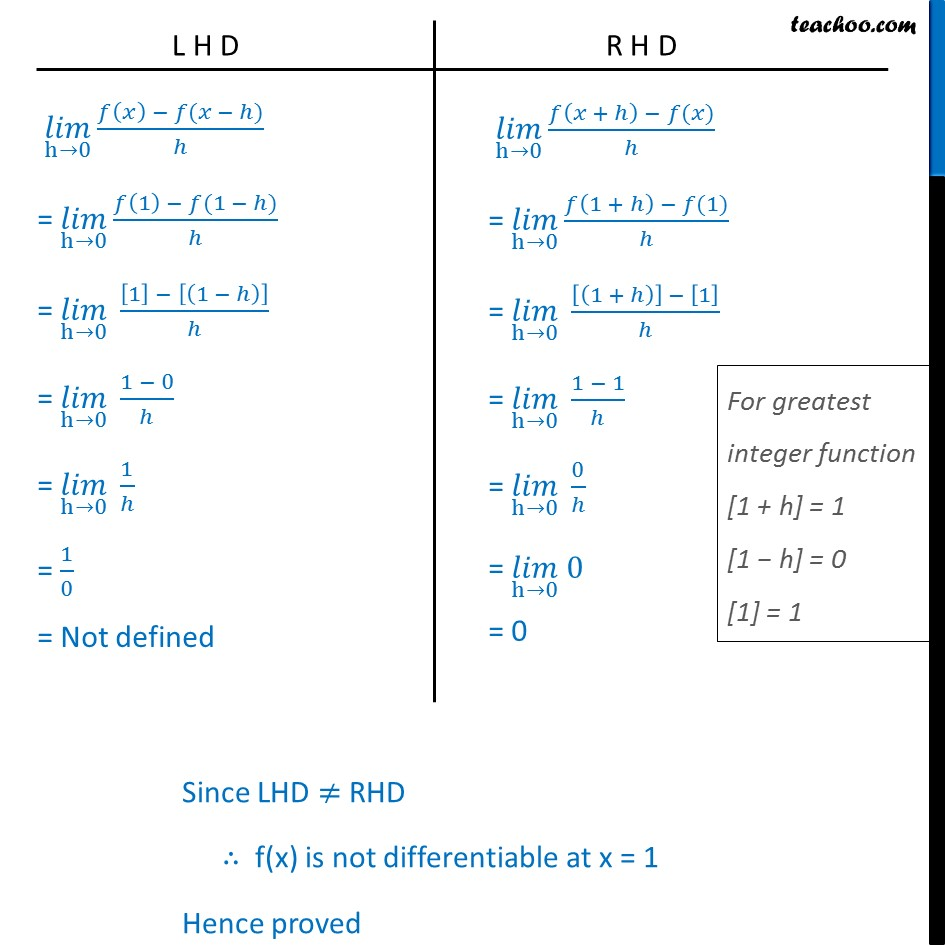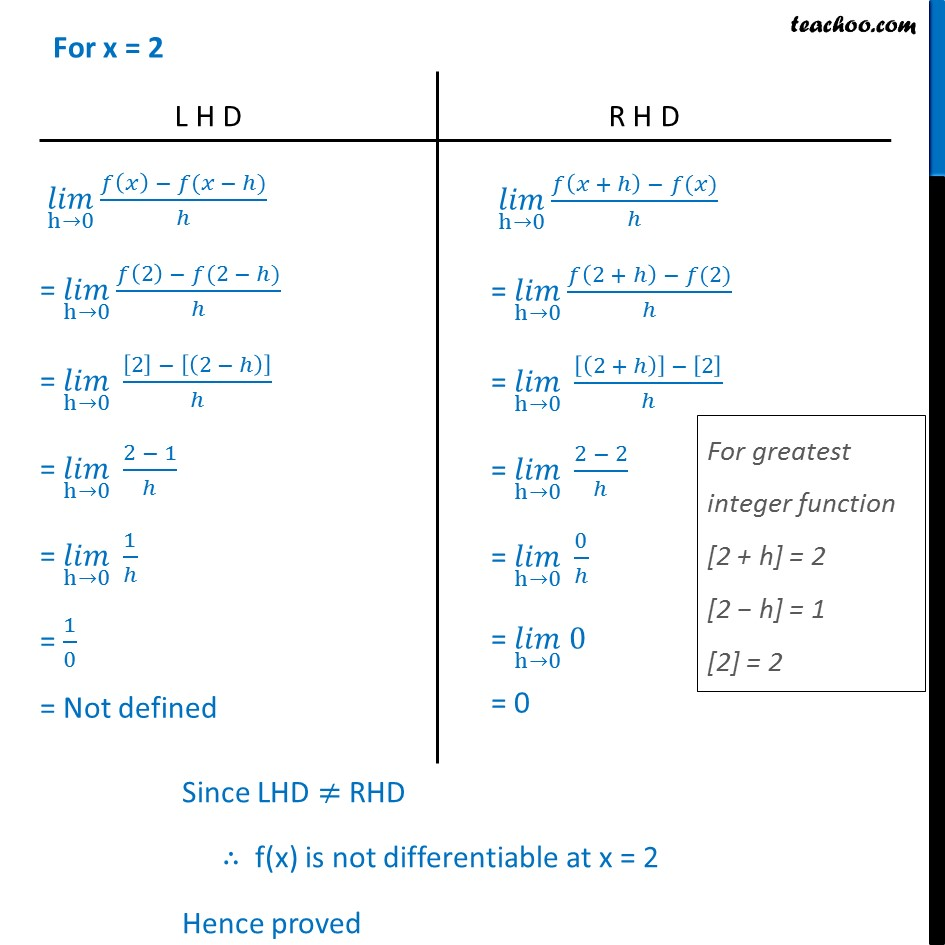1. Class 12
2. Important Question for exams Class 12
3. Chapter 5 Class 12 Continuity and Differentiability

Transcript

Ex 5.2, 10 (Introduction) Greatest Integer Function F(x) = [x] Example:  = 1 [1.2] = 1 [1.9999] = 1  = 2 [2.01] = 2  = 2 ∴ , [1 + h] = 1 [1 − h] = 0  = 1 Ex 5.2, 10 Prove that the greatest integer function defined by f (x) = [x], 0 < x < 3 is not differentiable at 𝑥=1 𝑎𝑛𝑑 𝑥= 2. f (x) = [x] At x = 1 f (x) is differentiable at x = 1 y if L H D = R H D (𝑙𝑖𝑚)┬(h→0) (𝑓(𝑥) − 𝑓(𝑥 − ℎ))/ℎ = (𝑙𝑖𝑚)┬(h→0) (𝑓(1) − 𝑓(1 − ℎ))/ℎ = (𝑙𝑖𝑚)┬(h→0) ( − [(1 − ℎ)])/ℎ = (𝑙𝑖𝑚)┬(h→0) (1 − 0)/ℎ = (𝑙𝑖𝑚)┬(h→0) 1/ℎ = 1/0 = Not defined (𝑙𝑖𝑚)┬(h→0) (𝑓(𝑥 + ℎ) − 𝑓(𝑥))/ℎ = (𝑙𝑖𝑚)┬(h→0) (𝑓(1 + ℎ) − 𝑓(1))/ℎ = (𝑙𝑖𝑚)┬(h→0) ([(1 + ℎ)] − )/ℎ = (𝑙𝑖𝑚)┬(h→0) (1 − 1)/ℎ = (𝑙𝑖𝑚)┬(h→0) 0/ℎ = (𝑙𝑖𝑚)┬(h→0) 0 = 0 Since LHD ≠ RHD ∴ f(x) is not differentiable at x = 1 Hence proved (𝑙𝑖𝑚)┬(h→0) (𝑓(𝑥) − 𝑓(𝑥 − ℎ))/ℎ = (𝑙𝑖𝑚)┬(h→0) (𝑓(2) − 𝑓(2 − ℎ))/ℎ = (𝑙𝑖𝑚)┬(h→0) ( − [(2 − ℎ)])/ℎ = (𝑙𝑖𝑚)┬(h→0) (2 − 1)/ℎ = (𝑙𝑖𝑚)┬(h→0) 1/ℎ = 1/0 = Not defined (𝑙𝑖𝑚)┬(h→0) (𝑓(𝑥 + ℎ) − 𝑓(𝑥))/ℎ = (𝑙𝑖𝑚)┬(h→0) (𝑓(2 + ℎ) − 𝑓(2))/ℎ = (𝑙𝑖𝑚)┬(h→0) ([(2 + ℎ)] − )/ℎ = (𝑙𝑖𝑚)┬(h→0) (2 − 2)/ℎ = (𝑙𝑖𝑚)┬(h→0) 0/ℎ = (𝑙𝑖𝑚)┬(h→0) 0 = 0 Since LHD ≠ RHD ∴ f(x) is not differentiable at x = 2 Hence proved

Chapter 5 Class 12 Continuity and Differentiability

Class 12
Important Question for exams Class 12